×

### Html & Html5

Introduction to Html

Html5 Grid

Html5 Graphics

Html5 Media

### If .. Else statements in Python

Before coming to the if.. else statement we should know about the operators, as we have discussed earlier also, but just recall some operators once again :-

Operators Description
a==b a equals to b
a!=b a not equals to b
a< b b is greater than a
a<=b a is less than or equals to b
a>b a is greater than b
a>=b a is greater or equals to b

Now these types of operators we mostly use in our program when there is a condition . These conditions can be used in many more ways but mostly we prefer the ‘if statements’ or loops . Ok let’s understand some of the conditions through our ‘if , else & elif’ statements :-

### if statement

‘if statement’ is used when in any particular condition we want to print something otherwise not . And we use ‘if’ keyword for this.
Let’s understand this by the following example :-

a = 100

b = 200

if b>a :

print("b is greater")

### Output of this Program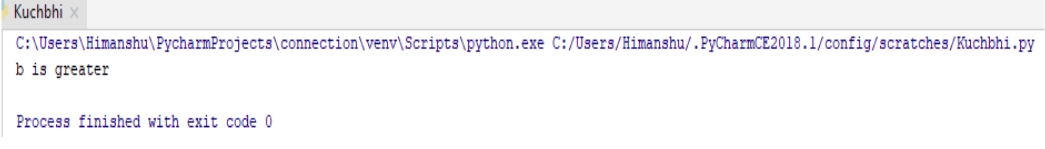So here in this program we assign some values to the variable ‘a’ & ‘b’ . Than through if statements we just gave command to our interpreter if ‘b’ is bigger than ‘a’ than print ‘b’ is greater , what it does.

The most important thing here is the indentation we use it after writing ‘if’ statement we use 4 spaces just to write the print command because python don’t use curly braces as other language does ,it only use indentation.

### elif statement

When , in any program there are many condition , and if our previous condition is not following true than through this ‘elif’ keyword our Python interpreter check new condition and if it is true than print it .
Let’s understand this by the following example :-

a = 200

b = 100

if b>a :

print("b is greater")

elif a>b :

print("a is greater")

### Output of this Program

Here in above program we just assign some values to the variables ‘a’ and ‘b’ . Than we just gave command to our Python interpreter that if the value of ‘a’ is greater than print “a is greater” otherwise print “b is greater” through the ‘elif’ keyword.
Let’s see what happens :-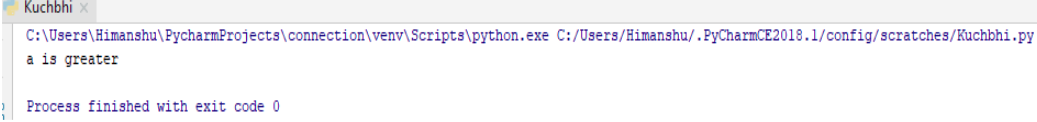So here it just gave us the correct output.

### else statement

When the condition which is in program does not match up or follows the true value than through ‘else’ keyword, we can print there as per we want .
Let’s understand this by the following example :-

a = 100

b = 100

if b>a :

print("b is greater")

elif a>b :

print("a is greater")

else:

print("Both are equals")

### Output of this Program

So in the above program we just assign some values to the variables ‘a’ and ‘b’ than through our Python interpreter we just gave command that if ‘a’ is greater print “a is greater than b” else print “b is greater than a” and else if these two conditions are not true than print “ Both are equals”.
Let’s see what happens :-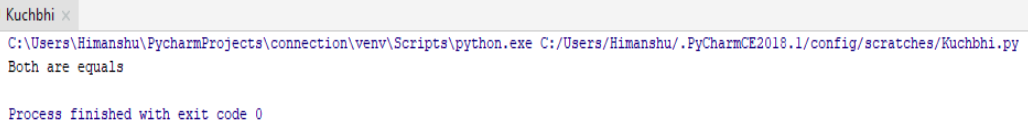So here it prints both are equals which is the correct one.

### Short hand if

As we have discussed about the ‘if’ statement , and also we used to understand that statement through example . That program is little bit lengthy too , but we can use of short hand if also .
Let’s understand this by the following example :-

a = 200

b = 100

if a>b :print("a is greater")

### Output of this Program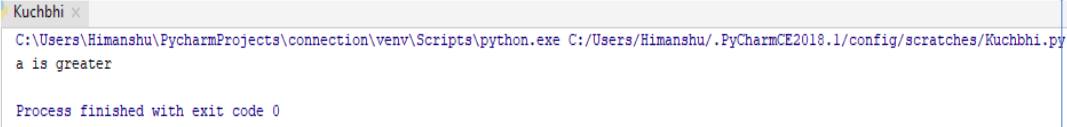So here also through this short hand if we have executed successfully to our program.

### Short hand if..else

As we have discussed about the ‘if’ & ‘else’ statement , and also we used to understand those statement through example . That program is little bit lengthy too , but we can use of short hand if else also .
Let’s understand this by the following example :-

a = 200

b = 100

print("a is greater") if a > b else print("both are equal") if a == b else print("b is greater ")

### Output of this ProgramSo here also through this short hand if.. else ,we have executed successfully to our program.

### and keyword

Through ‘and’ keyword we can combine our conditional statement.
Let’s understand this by the following example :-

a = 200

b = 100

c = 300

if a>b and c>a :

print("both conditions are ok")

### Output of this Program

In the above program , we just assign some values to our variables ‘a’ , ‘b’ & ‘c’ . Than we gave command to our Python interpreter that if ‘a is greater than b’ and ‘c is greater than a’ than only print “both conditions are ok” .
Let’s see what happens :-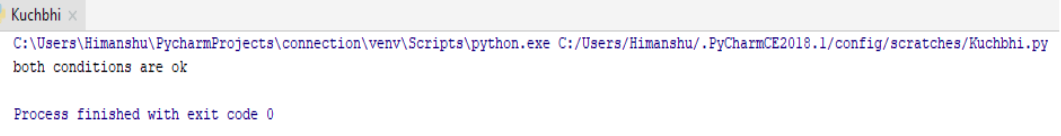So here through ‘and’ keyword we have checked our both condition.

### or keyword

Through ‘or’ keyword also we combine both the statement but through ‘or’ keyword we gives command that print the statement if any one condition from two or many is true .
Let’s understand this by the following example :-

a = 200

b = 200

c = 300

if a==b and c>a :

print("one is true")

### Output of this Program

In the above program , we just assign some values to our variables ‘a’ , ‘b’ & ‘c’ . Than we gave command to our Python interpreter that if ‘a is greater than b’ or ‘c is greater than a’ , if any one condition is also true from above than print “one is true” .
Let’s see what happens :-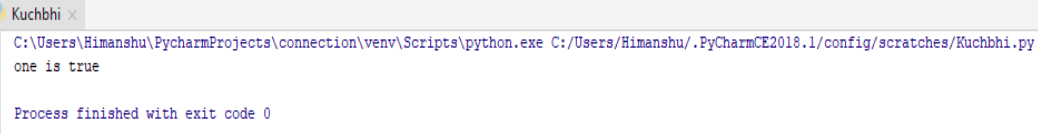So here through ‘or’ keyword we get our output which we want.

python training insitute| Best IT Training classes in Gurgaon | python training